# Chapter 2.2. Simple Calculations - Exam Problems

In the previous chapter, we got familiar with the system console and how to work with it – how to read numbers from the console and print output on the console. We went through the basic arithmetical operations and briefly mentioned data types. In this chapter, we will practice and consolidate what we have learned so far. We will solve a few more complicated problems given as exams problems.

## Reading Numbers from The Console

Before moving on to the problems, we will recall the most important of the material we have studied in the previous chapter. We will start with reading numbers from the console.

### Reading an Integer

We need to create a variable to store the number (for example num) and to use the standard command to read data from the console in combination with the function Integer.parseInt(…) which converts text into an integer:

int num = Integer.parseInt(scanner.nextLine());


### Reading a Floating-Point Number

We read a floating-point number the same way we read an integer, but this time we use the function Double.parseDouble(…):

double num = Double.parseDouble(scanner.nextLine());


## Printing Text Using Format Specifiers

The format specifier is an expression that will be replaced with a particular value while printing the output. The method System.out.printf(…) supports printing a string using format specifiers with the following format System.out.printf("format-string"[, arg1, arg2, … ]). As the first parameter of the method, we put the formatted string. As the second parameter of the method, we put so many arguments as the number of specifiers in the formatted string.

System.out.printf("You are %s %s, a %d-years old person from %s.",
firstName, lastName, age, town);


## Arithmetic Operators

Let us recall basic arithmetic operators for simple calculations.

### Operator +

int result = 3 + 5; // the result is 8


### Operator -

int result = 3 - 5; // the result is -2


### Operator *

int result = 3 * 5; // the result is 15


### Operator /

int result = 7 / 3; // the result is 2 (integer division)
double result2 = 5 / 2.0; // the result is 2.5 (fractional division)


## Concatenation

When using the operator + between string variables (or between a string and a number), the result is so-called concatenation (combining strings).

String firstName = "Ivan";
String lastName = "Ivanov";
int age = 19;
String str = firstName + " " + lastName + " is " + age + " years old";
// Ivan Ivanov is 19 years old


## Exam Problems

Now, after we have recalled how to make simple calculations, read and print numbers on the console, let's move to the problems. We will solve several problems from a SoftUni practical exam.

### Problem: Training Lab

A training lab has a rectangular size l and w meters without columns on the inside. The classroom is divided into two parts - left and right with a hallway located approximately in the middle of the room. In both parts, there are rows with desks. In the back of the hall, there is a big entrance door. In the front, there is a podium with a chair for the teacher. A single working place occupies 70 x 120 cm (a desk with a size 70 x 40 cm + space for a chair with a size 70 x 80 cm). The hallway has a width of at least 100 cm. It is known that because of the entrance door (which has an opening of 160cm) exactly one working space is lost and because of the podium (which has a size of 160 x 120 cm) is lost in exactly 2 working places. Write a program that reads the size of the classroom as input data and calculates the number of working places in it as it is described (look at the figure below).

### Input Data

As input data, we will read 2 numbers from the console, each on a separate line: l (length in meters) and w (width in meters).

Constraints: 3 ≤ w ≤ l ≤ 100.

### Output Data

Print on the console one integer number: the number of working places in the classroom.

### Sample Input and Output

Input Output Diagram
15
8.9
1298.4
5.2
39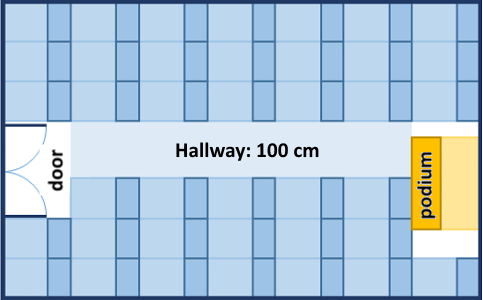#### Explanations to The Problems

In the first problem, the training lab length is 1500 cm. There could be located 12 rows (12 * 120 cm = 1440 + 60 cm residue). The training lab width is 890 cm. From them, 100 cm is for the hallway in the middle. In the rest 790 cm could be located 11 desks per row (11 * 70 cm = 770 cm + 20 cm residue). Number of working places = 12 * 11 - 3 = 132 - 3 = 129 (we have 12 rows with 11 working places = 132 minus 3 working places for podium and entrance door).

In the second problem, the training lab length is 840 cm. There could be located 7 rows (7 * 120 cm = 840, without residue). The training lab width is 520 cm. From them 100 cm are for the hallway in the middle. In the rest, 420 cm could be located 6 desks per row (6 * 70 cm = 420 cm, without residue). Number of working places = 7 * 6 - 3 = 42 - 3 = 39 (We have 7 rows with 6 working places per row = 42 minus 3 working places for podium and entrance door).

### Hints and Guidelines

Try to solve the problem on your own first. If you fail, use the Hints and Guidelines below.

#### An Idea for a Solution

In any programming problem, the important part is to build an idea for its solution before starting to write code. Let's carefully go through the problem requirements. We have to write a program that calculates the number of working places in a training lab where the number depends on the length and height of the room. We notice that the provided input data will be in meters, but we have the sizes for working places, and the hallway is in centimeters. To do the calculations, we must use the same measuring units no matter whether we choose to convert length and height into centimeters or the other data in meters. We use the first option for the presented solution.

Next, we have to calculate how many columns and how many rows with desks will fit in the training lab. We can calculate the columns by subtracting the width by the necessary space for the hallway (100 cm) and dividing the difference by 70 cm (the length of a working place). We will find the rows by dividing the length by 120 cm. In both operations, as a result, we can receive a number with integer and fractional parts. In a variable, we should store only the integer part. In the end, we multiply the number of rows by the number of columns and divide it by 3 (lost the working places because of the entrance door and podium), and we will receive the required value.

#### Choosing Data Types

From the example input data, it looks like we can get a number with integer and fractional parts. For that reason, it is not appropriate to choose the int data type. Therefore we will use double. Selecting the correct data type for the variables depends on the method we choose to solve the problem. Any programming problem as and this one also has more than one way to be solved. We will describe two methods.

#### Solution

It is time to go to the solution. We can divide it into three smaller problems:

• Read the input.
• Perform calculations.
• Print the output on the console.

The first thing we have to do is read the input from the console. With scanner.nextLine() we read the values from the console and with the function Double.parseDouble(…) we convert the string (text) value into double.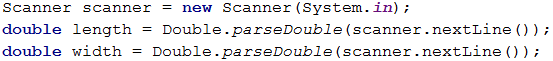Let’s move to the calculations. The special part here is that after dividing the numbers, we have to store only the integer part of the result in a variable.Search Google! Whenever we have an idea of how to solve a particular problem, but we do not know how to write it in Java, the easiest way to solve it is by looking for information on the net. If we are dealing with a problem that we assume that many other people have had before us, the easiest way to solve it is by looking for information on the Internet.

In this case, we can try with the following search: java gets the whole number part of double. We find out that one possible way is to use the method Math.floor(…). As the method works with a double data type, we create variables of the same type for the number of rows and columns.As a second solution, we can use: As we already know, the operator for division / operates differently on integers and floating-point numbers. When dividing integer with integer (for example int), the result is also an integer. Therefore, we can search for a way to convert the floating-point numbers of the height and the width of the training lab into integers and then divide them.

In this case, there could be data loss after removing the fractional part, so it is necessary for the conversion to be indicated explicitly (explicit typecasting). To indicate explicit conversion, we use the operator for converting data (type) by replacing the word type with the needed data type and placing it before the variable.With System.out.println(…) we print the result on the console.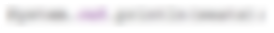### Testing in The Judge System

Test your solution here: https://judge.softuni.org/Contests/Practice/Index/650#0.

### Problem: Vegetable Market

A gardener sells the harvest from his garden on the vegetable market. He sells vegetables for N BGN per kilogram and fruits for M BGN per kilogram. Write a program to calculate the earnings from the harvest in EUR (assuming that one EUR is equal to BGN 1.94).

### Input Data

As input data, we will read 4 numbers from the console, each on a separate line:

• On the first line – the price of the vegetables, per kilogram – a floating-point number.
• On the second line – the price of the fruits, per kilogram – a floating-point number.
• On the third line – total kilograms of vegetables – an integer.
• On the fourth line – total kilograms of fruits – an integer.

Constraints: all numbers will be in the range of 0.00 to 1000.00.

### Output Data

Print on the console one floating-point number: the earnings of all fruits and vegetables in EUR.

### Sample Input and Output

Input Output Input Output
0.194
19.4
10
10
101 1.5
2.5
10
10
20.6185567010309

Explanations to the first problem:

• The vegetables cost: 0.194 BGN * 10 kg. = 1.94 BGN
• The fruits cost: 19.4 BGN * 10 kg. = 194 BGN
• Total: 195.94 BGN = 101 EUR.

### Hints and Guidelines

First, we will give a few ideas and particular hints for solving the problem, followed by the essential part of the code.

#### An Idea for a Solution

Let's first go through the problem requirements. In this case, we have to calculate the total income from the harvest. It is equal to the sum of the profit from the fruits and vegetables, which we can calculate by multiplying the price per kilogram by the quantity. Our input data is in leva, and the output should be in EUR. According to the condition 1 Euro equals 1.94lv, so to obtain the requested output value, we have to divide the sum by 1.94.

#### Choosing Data Types

After we have a clear idea of how to solve the problem, we can proceed to choose appropriate data types. Let's go through the input: we have two integers for total kilograms of vegetables and fruits, so the variables we declare to store their values will be of int. The prices of the fruits and vegetables are said to be floating-point numbers, so the variables will be of type double.

We can also declare two variables to store the income from the fruits and vegetables separately. As we are multiplying a variable of type int (total kilograms) with another of double type (price), the result should also be of type double. Let's clarify that generally, operators work with arguments of the same type. Therefore, to multiply values of different data types, we have to convert them into the same type. When there are different types in one expression, conversion is done to the highest type, in this case, in this case double. Because this conversion does not suggest data loss the conversion is implicit and is automatically done by the compiler.

The output should also be a floating-point number. This means that the result will be stored in a variable of type double.

#### Solution

It is time to move on to the solution. We can mentally divide it into three subproblems:

• Read the input.
• Perform calculations.
• Print the output on the console.

To read the input data, we declare variables, being careful to name them in such a way that it tells us what values the variables contain. With scanner.nextLine() we read values from the console and with the functions Integer.parseInt(…) and Double.parseDouble(…) we convert the particular string value into int and double.We do the necessary calculations:The problem does not specify a special output format, so we just need to calculate the required value and print it on the console. As in mathematics and so in programming, the division has a priority over addition. However, in this problem first, we have to calculate the sum of the two input values, and then we have to divide by 1.94. To give priority to the addition, we can use brackets. With System.out.println(…), we print the output on the console.### Testing in The Judge System

Test your solution here: https://judge.softuni.org/Contests/Practice/Index/650#1.

### Problem: Change Tiles

On the ground in front of an apartment building tiles need to be placed. The place is square with a side of N meters. The tiles are W meters wide and L meters long. There is a bench in the area with a width of M meters and a length of O meters. There is no need to put tiles under it. To place each tile requires 0.2 minutes.

Write a program that reads from the console the dimensions of the ground, the tiles, and the bench. Then calculates how many tiles are needed to cover the place and calculates the time to place all the tiles.

Example: A ground with a size 20 m. has an area of 400 sq.m. A bench that is 1 m. wide and 2 m. long has an area of 2 sq.m. One tile is 5 m. wide and 4 m. long and has an area of = 20 sq.m. The area that needs to be covered is 400 - 2 = 398 sq.m. The tiles that are needed are 398 / 20 = 19.90. The needed time is 19.90 * 0.2 = 3.98 minutes.

### Input Data

As input data, we will read 5 numbers from the console, each on a separate line:

• N – the length of a side of the ground within the range of [1 … 100].
• W – the width of a tile in the range of [0.1 … 10.00].
• L – the length of a tile in the range of [0.1 … 10.00].
• M – the width of the bench in the range of [0 … 10].
• O – the length of the bench in the range of [0 … 10].

### Output Data

Print on the console two numbers, each on a new line: number of tiles needed for the repair and the time for placing them.

## Sample Input and Output

Input Output Input Output
20
5
4
1
2
19.9
3.98
40
0.8
0.6
3
5
3302.08333333333
660.416666666667

Explanation of the problem:

• Total area = 20 * 20 = 400.
• Bench area = 1 * 2 = 2.
• Coverage area = 400 – 2 = 398.
• Tile area = 5 * 4 = 20.
• Required tiles = 398 / 20 = 19.9.
• Time required = 19.9 * 0.2 = 3.98.

### Hints and Guidelines

Let's make a drawing to clarify the problem requirements. It can look like this: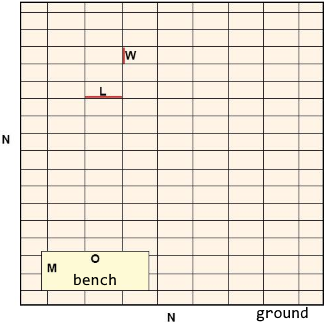### An Idea for a Solution

We need to calculate the number of tiles to cover the ground and the time for placing them. To calculate the number of tiles, we have to calculate the area that needs to be covered and divide it by the area per tile. The ground is square, and we find the total area using the formula N * N. After that, we calculate the area of the bench by multiplying its two sides M * O. After that, to obtain the area that needs to be covered, we subtract the area of the bench from the area of the ground.

We calculate the area of a single tile by multiplying its two sides with one another - W * L. As we already said, now we have to divide the area to the cove by the area of a single tile. In this way, we find the number of necessary tiles, which we multiply by 0.2 (the time needed for changing a single tile). Now, we have the required output.

### Choosing Data Types

Input data for the length of the side of the ground, the width, and the length of the bench are integer numbers, so to keep their values, we will declare variables of type int. For the width and the length of the tiles we will read floating-point numbers, so we will use variables of type double. The output will be a floating-point number, so used variables will be of double type.

### Solution

As in the previous problems, we can divide the solution into three subproblems:

• Read the input.
• Perform calculations.
• Print the input on the console.

The first thing we have to do is go through the input data of the problem. It is important to pay attention to the sequence of reading input data. With scanner.nextLine() we read values from the console and with Integer.parseInt(…) and Double.parseDobule(…) we convert the particular string value into int and double.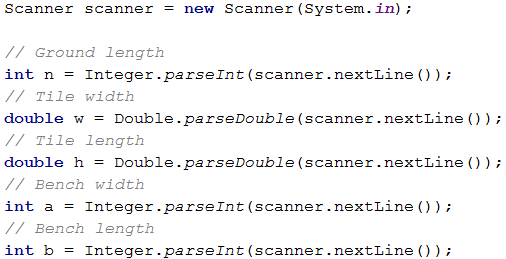Once we have initialized the variables and saved the corresponding values in them, we proceed to the calculations. As the values of the variables n, a, and b that we work with are stored in variables of type int, we can also declare for the results variables of the same type.The variables w and h are of type double, so for the area of a single tile, we create a variable of the same type. Finally, we calculate the values that we have to print on the console. The number of needed tiles we get by dividing the area that needs to be covered by the area of a single tile. When dividing two numbers, one of which is the floating-point number a result is also a floating-point number. For the calculations to be correct we store the result in a variable of type double. The problem does not specify special formatting or rounding of the output, so we just print the values with System.out.println(…).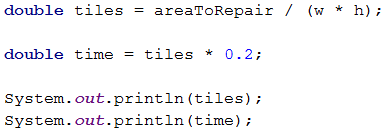### Testing in The Judge System

Test your solution here: https://judge.softuni.org/Contests/Practice/Index/650#2.

### Problem: Money

Some time ago Pesho bought bitcoins. He plans to go on a trip around Europe and he will need euros. Besides bitcoins, he has Chinese yuan(CNY). Pesho wants to exchange his money in euros for the trip. Write a program that calculates how much euros he can buy depending on the following exchange rates:

• 1 Bitcoin = 1168 BGN
• 1 CNY = 0.15 USD
• 1 USD = 1.76 BGN
• 1 EUR = 1.95 BGN

The exchange office has a commission within 0 to 5 percent from the final amount in EUR.

### Input Data

As input data, we will read 3 numbers from the console, each on a separate line:

• On the first line – a number of Bitcoins. Integer in the range of [0 … 20].
• On the second line – a number of Chinese yuan. Floating-point number in the range of [0.00 … 50 000.00].
• On the third line – commission fee. Floating-point number in the range of [0.00 … 5.00].

### Output Data

Print the result of the exchange of currencies on the console. Rounding the result is not necessary.

### Sample Input and Output

Input Output Input Output Input Output
1
5
5
569.668717948718 20
5678
2.4
12442.2442010256 7
50200.12
3
10659.4701177436

Explanation:

• 1 Bitcoin = 1168 BGN
• 5 CNY = 0.75 USD
• 0.75 USD = 1.32 BGN
• 1168 + 1.32 = 1169.32 BGN =599.651282051282 EUR
• Commission fee: 5% of 599.651282051282 = 29.9825641025641
• Result: 599.651282051282 - 29.9825641025641 = 569.668717948718 EUR

### Hints and Guidelines

Let's think again about how we can solve the problem before we start writing code.

#### An Idea for a Solution

We see that the number of bitcoins and the number of Chinese yuans will be given in the input. The output should be in euros. The exchange rates that we have to work with are in the problem condition. We notice that we can only exchange the sum in BGN to EUR, so we have first to calculate the whole sum that Peter has in BGN and then to calculate the output.

As we have information for the exchange rate of Bitcoins to BGN, we can directly exchange them. On the other hand, to get the value of Chinese yuans in BGN, first, we have to exchange them in USD and then USD to BGN. Finally, we will sum the two values and calculate how much EUR that is.

To calculate the commission fee and subtract the new sum from the total one. The commission is a floating-point number, representing a percentage of the total sum. Let's divide it from the beginning by 100 to calculate its percentage value. We will multiply the percentage value by the sum in EUR, and the result divides with the same sum and print the final sum on the console.

#### Choosing Data Types

Bitcoins are given as an integer, and for their value, we can declare a variable of type int. As a number Chinese yuan and commission fee, we will obtain a floating-point number, so we will use double. A double is the data type with a bigger size and the output should also be a floating-point number, we will use it for the other variables we create.

#### Solution

After we have built an idea on how to solve the problem and we have chosen the data types, it is time to get to write the code. As in the previous problems, we can divide the solution into three smaller problems:

• Read the input.
• Perform calculations.
• Print the input on the console.

We declare the variables that we are going to use and again we carefully have to choose meaningful names in such a way that it tells us what values the variables contain. We initialize their values: with scanner.nextLine(), we read the input numbers from the console and convert the string entered by the user to int or double.We do the necessary calculations: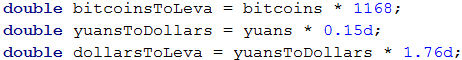Finally, we calculate the commission fee value and subtract it from the sum in EUR. Let's pay attention to this: euro -= commission * euro. It is the shortcut to write euro = euro - (commission * euro). In this case, we use a combined assignment operator -=, which subtracts the right value of the operand from the one to the left. The operator for multiplication * has a higher priority than the combined operator than the expression commission * euro is performed first. Then its value is subtracted from the value to the left of the operand.

The problem does not specify special formatting or rounding of the result, so we just have to calculate the output and print it on the console.Let's pay attention to something that applies to all other problems of this type: written like that, the solution of the problem is pretty detailed. As the problem is not too complex, we could write one complex expression, where after we read the input data, we calculate the output. For example, such an expression would look like this:This code would print a correct result, but it is hard to read. It won't be easy to find out how it works, and whether it contains any mistakes, as well as finding such and correcting them. It is better to instead of one complex expression, write a few simpler ones and store their values in variables with appropriate names. This way, the code is cleaner and easily maintainable.

## Testing in The Judge System

Test your solution here: https://judge.softuni.org/Contests/Practice/Index/650#3.

## Problem: Daily Earnings

Ivan is a programmer in an American company and he works from home N days per month and earns M USD per day. At the end of the year, Ivan gets a bonus which equals 2.5 monthly salaries. From what he earned during the year, the withholding tax is 25%. Write a program that calculates the amount of Ivan's net average earning in BGN per day because he spends it in Bulgaria. We accept that one year has exactly 365 days. The exchange rate of USD to BGN will be read from the console.

### Input Data

As input data, we will read 3 numbers from the console, each on a separate line:

• On the first line – working days of the month. Integer in the range of [5 … 30].
• On the second line – money earned per day. Floating-point number in the range of [10.00 … 2000.00].
• On the third line – exchange rate USD to BGN /1 USD = X BGN/. Floating-point number in the range of [0.99 … 1.99].

### Output Data

On the console print average earning in BGN per day. The result should be rounded up to the second digit after the decimal point.

### Sample Input and Output

Input Output Input Output Input Output
21
75.00
1.59
74.61 15
105
1.71
80.24 22
199.99
1.50
196.63

Explanation:

• Monthly salary = 21 * 75 = 1575 USD.
• Annual income = 1575 * 12 + 1575 * 2.5 = 22837.5 USD.
• Taxes = 25% from 22837.5 = 5709.375 USD.
• Net annual income = 17128.125 dollars = 27233.71875 BGN.
• Average earning per day = 27233.71875 / 365 = 74.61 BGN.

### Hints and Guidelines

Firstly, we have to analyze the problem and think of a way to solve it. Then we will choose data types, and finally, we will write the code.

#### An Idea for a Solution

Let's first calculate how much the monthly salary of Ivan is. We will do this by multiplying the working days per month by the money he earns per day. We multiply the result by 12 to calculate his yearly salary and then multiply his yearly salary by 2.5 to calculate the bonus. Adding the two values obtained, we will calculate his total annual income. We have to subtract 25% from it for taxes. We can do this by multiplying the total income by 0.25 and subtracting the result from it. Then we exchange the USD to BGN and divide the result by the days of a year, which we assume are 365.

#### Choosing Data Types

The working days per month are given as an integer, so we can declare a variable of type int. For the earned money and the exchange rate of USD to BGN, we will obtain a floating-point number, so for them, we use double as type. A double is a type with a bigger size and the output should also be a floating-point number, we use double for the other variables we create.

#### Solution

After we have an idea of how to solve the problem, and we have considered the data types that we will use, we can start writing the code. As in the previous problems, we can divide the solution into three smaller problems:

• Read the input.
• Perform calculations.
• Print the output on the console.

We declare the variables that we are going to use by trying to choose meaningful names. With scanner.nextLine() we read the input numbers from the console and we convert the input string to int or double with Integer/Double.parseDouble/Double(…).We do the necessary calculations: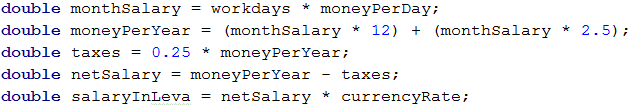We could write an expression that calculates the annual income without brackets as well. Multiplication is an operation that has a higher priority over addition and performs first. Despite that, it is a good practice to use brackets when using more operators because the code becomes more easily readable and the chances of making a mistake are smaller.

Finally, we have to print the result on the console. We notice that the number has to be rounded up to the second digit after the decimal point. For this purpose, we can use formatted specifiers, an item that will be replaced by a particular value when printing. In Java, for formatting specifier is used % followed by a specific letter (specifier). We can format an integer or a floating-point number by using F/f, or D/d. In the case of a floating-point number, before the letter, we put a dot and a whole positive number specifying the number of digits after the decimal point.

The final result is as follows: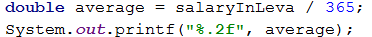### Testing in The Judge System

Test your solution here: https://judge.softuni.org/Contests/Practice/Index/650#4.